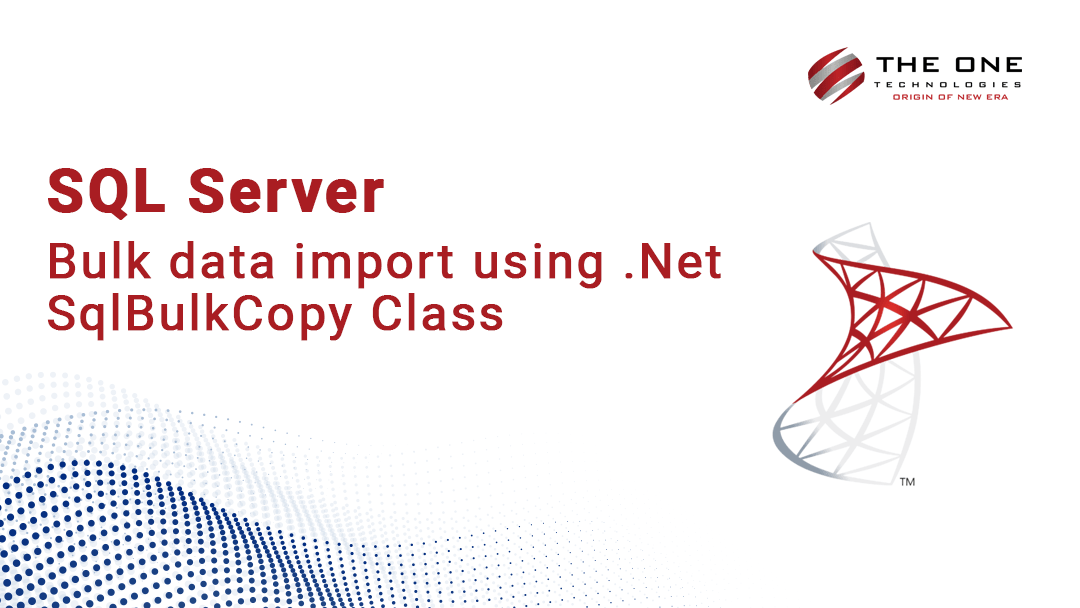# SQL Server - Bulk data import using .Net SqlBulkCopy Class## 1. Create table in database (skip this step if you can use existing table)

``````CREATE TABLE [dbo].[ProductOrder](
[ProductOrderID] [int] IDENTITY(1,1) NOT NULL,
[OrderID] [int] NULL,
[Value] [int] NULL,
[Length] [int] NULL,
[TotalVolume] [decimal](18, 2) NULL,
CONSTRAINT [PK_ProductOrder] PRIMARY KEY CLUSTERED
(
[ProductOrderID] ASC
)WITH (PAD_INDEX  = OFF, STATISTICS_NORECOMPUTE  = OFF, IGNORE_DUP_KEY = OFF, ALLOW_ROW_LOCKS  = ON, ALLOW_PAGE_LOCKS  = ON) ON [PRIMARY]
) ON [PRIMARY]

GO``````

## 2. C#.net code

2.1 Read data in DataTable from any source(excel, csv etc..)
2.2 Create instance of SqlBulkCopy class and map DataTable columns to Database table
2.3 Use WriteToServer method of SqlBulkCopy class to load data into Database

``````private void DataImport()
{
//Creates a datatable and add few dummy records
DataTable orderDetail = new DataTable("ItemDetail");

DataRow dr = orderDetail.NewRow();
dr["ID"] = 1;
dr["value"] = 50;
dr["length"] = 5;

dr = orderDetail.NewRow();
dr["ID"] = 2;
dr["value"] = 60;
dr["length"] = 15;

using (SqlConnection cn = new SqlConnection(connect))
using (SqlBulkCopy bcp = new SqlBulkCopy(cn))
{
cn.Open();
//Column mapping..
bcp.DestinationTableName = "ProductOrder";//Here 'ProductOrder' is database table name, Make sure have 'ProductOrder' into database
bcp.ColumnMappings.Add("ID", "OrderID"); //Here 'ID' is a datatable column name and 'OrderID' is a sql table columname
bcp.ColumnMappings.Add("value", "Value");//Here 'value' is a datatable column name and 'Value' is a sql table columname
bcp.ColumnMappings.Add("length", "Length");//Here 'length' is a datatable column name and 'Length' is a sql table columname